# Binomial probabilities on the TI 83 or 84 calculator

In this article, we will learn how to find binomial probabilities using your TI 83 or 84 calculator. We’re going to assume that you already know how to determine whether or not a probability experiment is binomial and instead just focus on how to use the calculator itself.

There are two functions you will need to use, and each is for a different type of problem. We will look at each of them below, using an example.

## binomialpdf

The binompdf function on your calculator is for finding the probability of exactly some number of successes.### Example

A survey determines that 62% of the attendees at a conference have attended a similar conference in the last year. Suppose that 9 attendees are randomly selected. Find the probability that exactly 4 have attended a similar conference in the last year.

Let X represent the number of attendees that have attended a similar conference in the last year. We are then trying to find:

P(X = 4)

#### Step 1: Go to the distributions menu on the calculator and select binompdf.

To get to this menu, press:followed byScroll down to binompdf near the bottom of the list.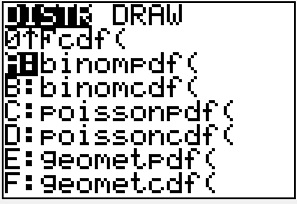Press enter to bring up the next menu.

 TI83 TI84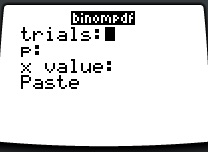#### Step 2: Enter the required data.

In this problem, there are 9 people selected (n = number of trials = 9). The probability of success is 0.62 and we are finding P(X = 4). How you enter this looks different in each calculator.

 TI83 TI84 Type in 9, 0.62, 4) and then press enter. It will always be in this order: binompdf(n, p, c).Fill in the needed information, highlight paste, and then press enter. You will then need to press enter again to get the final answer.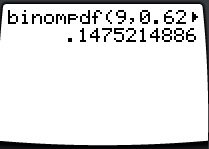This shows that the probability of exactly 4 successes is about 0.1475. So, the probability that out of the 9 people we selected, exactly 4 have attended a similar conference in the last year is 0.1475.

## binomialcdf

The “cdf” in this stands for cumulative. This function will take whatever value we type in, and find the cumulative probability for that value and all the values below it. In other words, this function allows us to calculate the probability of “c or fewer” successes, for some number c.### Example

A survey determines that 62% of the attendees at a conference have attended a similar conference in the last year. Suppose that 9 attendees are randomly selected. Find the probability that 6 or fewer of these attendees have attended a similar conference in the last year.

This is the same example we used before, but now we are finding a different probability. So, we will once again let X represent the number of attendees that have attended a similar conference in the last year. We are now trying to find:

P(X ≤ 6)

This is the type of probability that the binomcdf function is built for!

#### Step 1: Go to the distributions menu on the calculator and select binomcdf.

To get to this menu, press:followed byScroll down to binomcdf near the bottom of the list.Press enter to bring up the next menu.

 TI83 TI84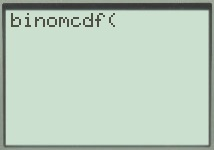#### Step 2: Enter the required data.

In this problem, there are 9 people selected (n = number of trials = 9). The probability of success is 0.62 and we are finding P(X ≤ 6). How you enter this looks different in each calculator.

 TI83 TI84 Type in 9, 0.62, 6) and then press enter. It will always be in this order: binomcdf(n, p, c).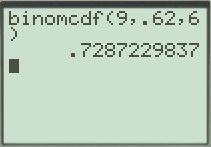Fill in the needed information, highlight paste, and then press enter. You will then need to press enter again to get the final answer.This shows that the probability of 6 or fewer successes is about 0.7287. This means that out of the 9 people we randomly selected, the probability that 6 or fewer have attended a similar conference in the last year is 0.7287.